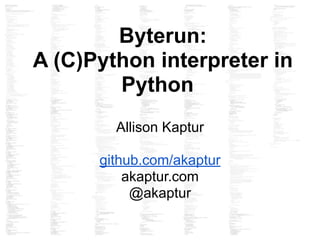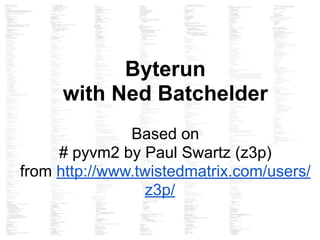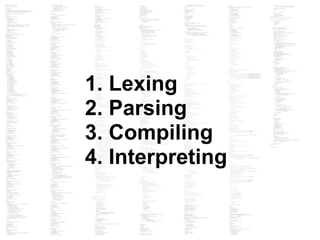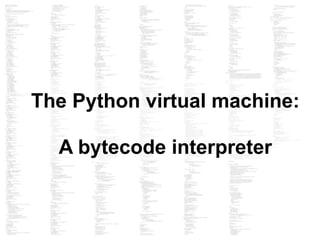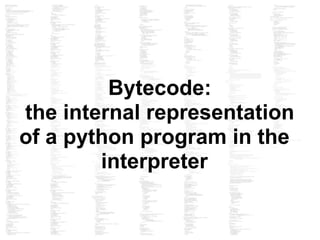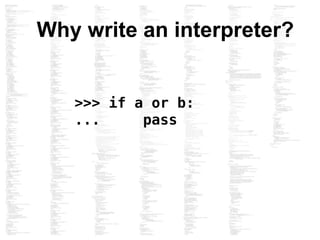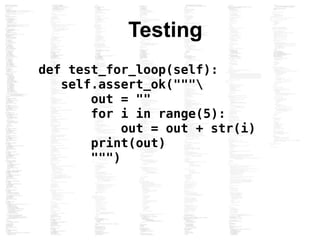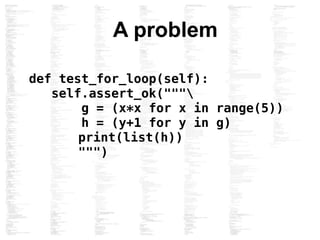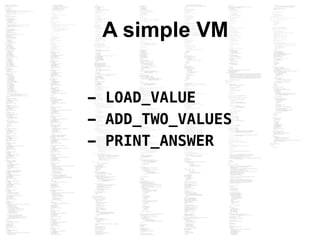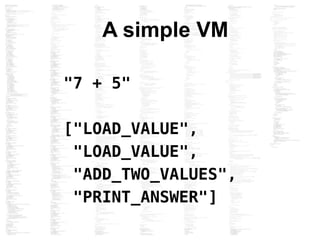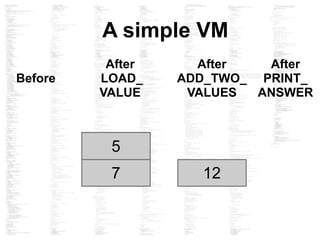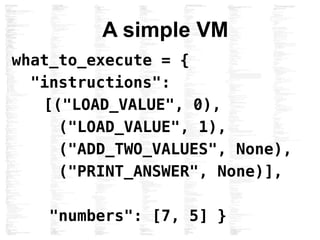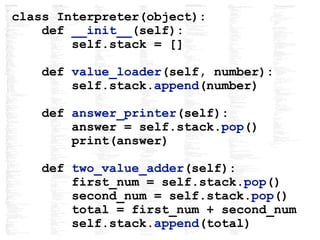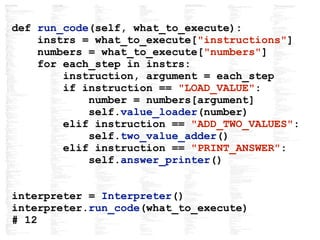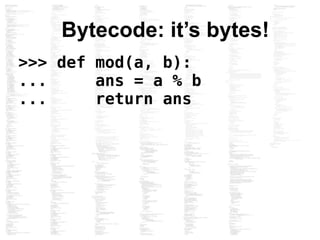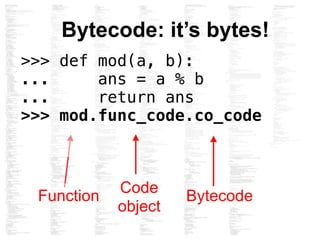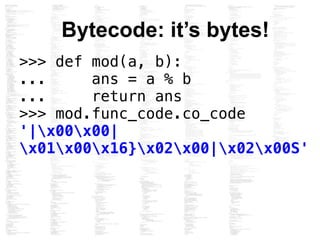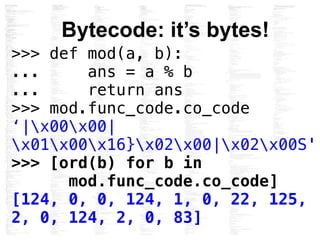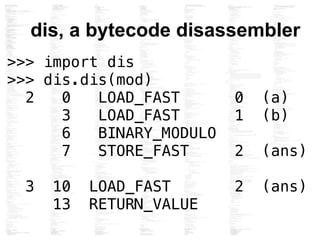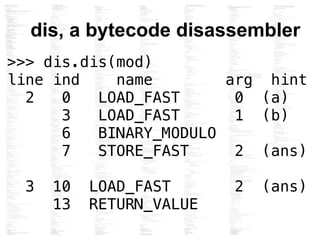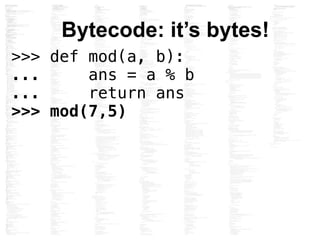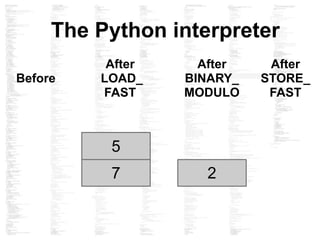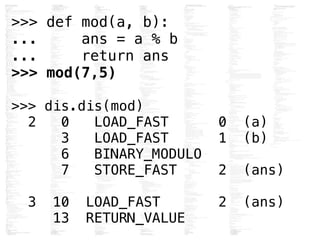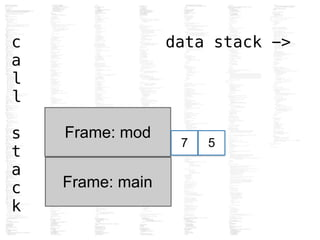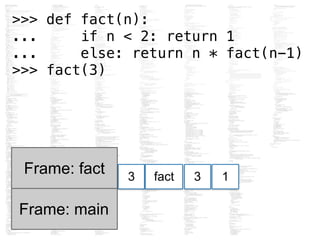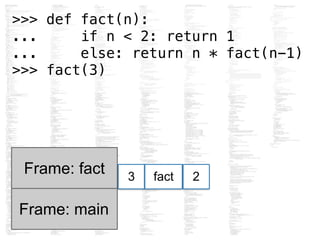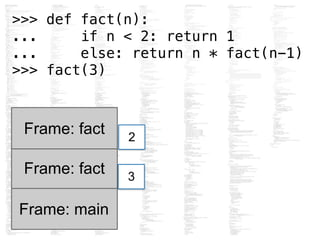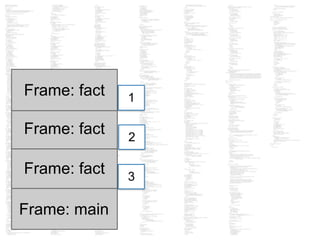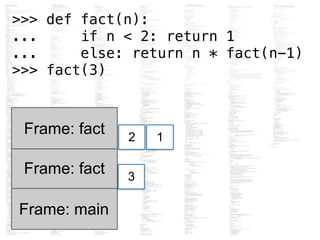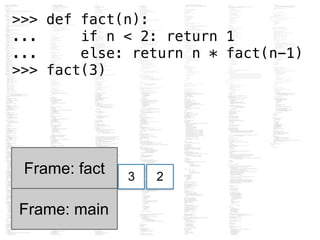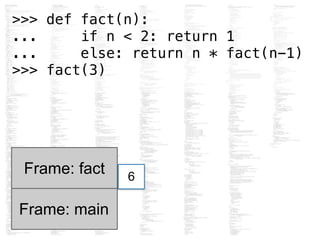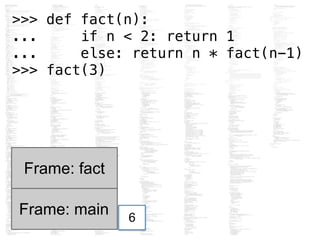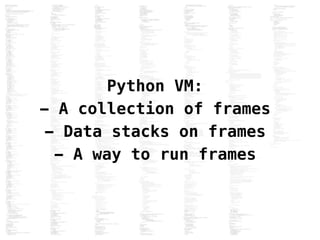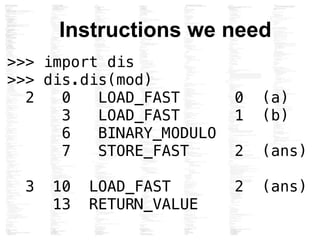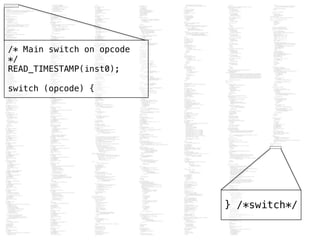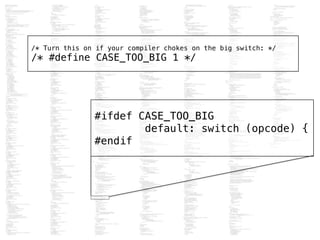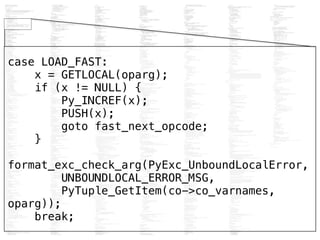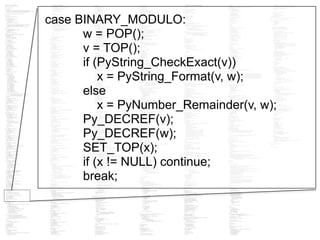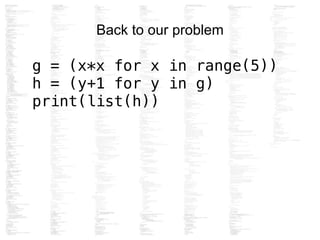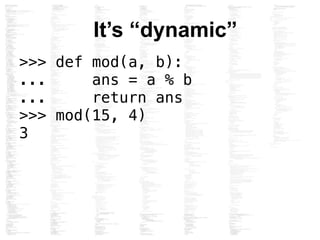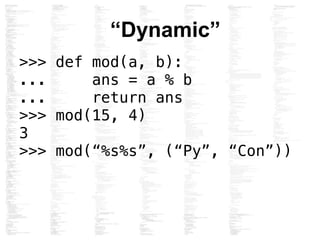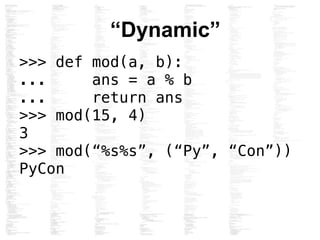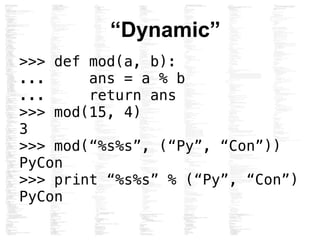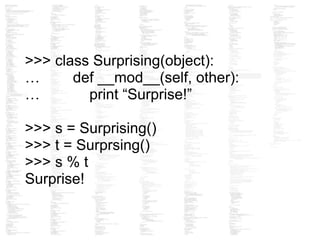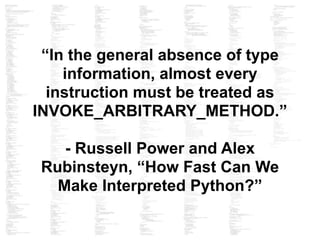1 of 51

### Bytes in the Machine: Inside the CPython interpreter

1. Byterun: A (C)Python interpreter in Python Allison Kaptur github.com/akaptur akaptur.com @akaptur
2. Byterun with Ned Batchelder Based on # pyvm2 by Paul Swartz (z3p) from http://www.twistedmatrix.com/users/ z3p/
3. “Interpreter”
4. 1. Lexing 2. Parsing 3. Compiling 4. Interpreting
5. The Python virtual machine: A bytecode interpreter
6. Bytecode: the internal representation of a python program in the interpreter
7. Why write an interpreter? >>> if a or b: ... pass
8. Testing def test_for_loop(self): self.assert_ok(""" out = "" for i in range(5): out = out + str(i) print(out) """)
9. A problem def test_for_loop(self): self.assert_ok(""" g = (x*x for x in range(5)) h = (y+1 for y in g) print(list(h)) """)
12. 7 5 12 Before After ADD_TWO_ VALUES After LOAD_ VALUE A simple VM After PRINT_ ANSWER
14. class Interpreter(object): def __init__(self): self.stack = [] def value_loader(self, number): self.stack.append(number) def answer_printer(self): answer = self.stack.pop() print(answer) def two_value_adder(self): first_num = self.stack.pop() second_num = self.stack.pop() total = first_num + second_num self.stack.append(total)
15. def run_code(self, what_to_execute): instrs = what_to_execute["instructions"] numbers = what_to_execute["numbers"] for each_step in instrs: instruction, argument = each_step if instruction == "LOAD_VALUE": number = numbers[argument] self.value_loader(number) elif instruction == "ADD_TWO_VALUES": self.two_value_adder() elif instruction == "PRINT_ANSWER": self.answer_printer() interpreter = Interpreter() interpreter.run_code(what_to_execute) # 12
16. Bytecode: it’s bytes! >>> def mod(a, b): ... ans = a % b ... return ans
17. Bytecode: it’s bytes! Function Code object Bytecode >>> def mod(a, b): ... ans = a % b ... return ans >>> mod.func_code.co_code
18. Bytecode: it’s bytes! >>> def mod(a, b): ... ans = a % b ... return ans >>> mod.func_code.co_code '|x00x00| x01x00x16}x02x00|x02x00S'
19. Bytecode: it’s bytes! >>> def mod(a, b): ... ans = a % b ... return ans >>> mod.func_code.co_code ‘|x00x00| x01x00x16}x02x00|x02x00S' >>> [ord(b) for b in mod.func_code.co_code] [124, 0, 0, 124, 1, 0, 22, 125, 2, 0, 124, 2, 0, 83]
20. dis, a bytecode disassembler >>> import dis >>> dis.dis(mod) 2 0 LOAD_FAST 0 (a) 3 LOAD_FAST 1 (b) 6 BINARY_MODULO 7 STORE_FAST 2 (ans) 3 10 LOAD_FAST 2 (ans) 13 RETURN_VALUE
21. dis, a bytecode disassembler >>> dis.dis(mod) line ind name arg hint 2 0 LOAD_FAST 0 (a) 3 LOAD_FAST 1 (b) 6 BINARY_MODULO 7 STORE_FAST 2 (ans) 3 10 LOAD_FAST 2 (ans) 13 RETURN_VALUE
22. Bytecode: it’s bytes! >>> def mod(a, b): ... ans = a % b ... return ans >>> mod(7,5)
23. 7 5 2 Before After BINARY_ MODULO After LOAD_ FAST The Python interpreter After STORE_ FAST
24. >>> def mod(a, b): ... ans = a % b ... return ans >>> mod(7,5) >>> dis.dis(mod) 2 0 LOAD_FAST 0 (a) 3 LOAD_FAST 1 (b) 6 BINARY_MODULO 7 STORE_FAST 2 (ans) 3 10 LOAD_FAST 2 (ans) 13 RETURN_VALUE
25. c data stack -> a l l s t a c k Frame: main Frame: mod 7 5
26. Frame: main Frame: fact >>> def fact(n): ... if n < 2: return 1 ... else: return n * fact(n-1) >>> fact(3) 3 3fact 1
27. Frame: main Frame: fact >>> def fact(n): ... if n < 2: return 1 ... else: return n * fact(n-1) >>> fact(3) 3 2fact
28. Frame: main Frame: fact >>> def fact(n): ... if n < 2: return 1 ... else: return n * fact(n-1) >>> fact(3) 3 Frame: fact 2
29. Frame: main Frame: fact 3 Frame: fact 2 Frame: fact 1
30. Frame: main Frame: fact 3 Frame: fact 2 1 >>> def fact(n): ... if n < 2: return 1 ... else: return n * fact(n-1) >>> fact(3)
31. Frame: main Frame: fact 3 2 >>> def fact(n): ... if n < 2: return 1 ... else: return n * fact(n-1) >>> fact(3)
32. Frame: main Frame: fact 6 >>> def fact(n): ... if n < 2: return 1 ... else: return n * fact(n-1) >>> fact(3)
33. Frame: main Frame: fact 6 >>> def fact(n): ... if n < 2: return 1 ... else: return n * fact(n-1) >>> fact(3)
34. Python VM: - A collection of frames - Data stacks on frames - A way to run frames
35. >>> import dis >>> dis.dis(mod) 2 0 LOAD_FAST 0 (a) 3 LOAD_FAST 1 (b) 6 BINARY_MODULO 7 STORE_FAST 2 (ans) 3 10 LOAD_FAST 2 (ans) 13 RETURN_VALUE Instructions we need
36. } /*switch*/ /* Main switch on opcode */ READ_TIMESTAMP(inst0); switch (opcode) {
37. #ifdef CASE_TOO_BIG default: switch (opcode) { #endif /* Turn this on if your compiler chokes on the big switch: */ /* #define CASE_TOO_BIG 1 */
38. Instructions we need >>> import dis >>> dis.dis(mod) 2 0 LOAD_FAST 0 (a) 3 LOAD_FAST 1 (b) 6 BINARY_MODULO 7 STORE_FAST 2 (ans) 3 10 LOAD_FAST 2 (ans) 13 RETURN_VALUE
39. case LOAD_FAST: x = GETLOCAL(oparg); if (x != NULL) { Py_INCREF(x); PUSH(x); goto fast_next_opcode; } format_exc_check_arg(PyExc_UnboundLocalError, UNBOUNDLOCAL_ERROR_MSG, PyTuple_GetItem(co->co_varnames, oparg)); break;
40. case BINARY_MODULO: w = POP(); v = TOP(); if (PyString_CheckExact(v)) x = PyString_Format(v, w); else x = PyNumber_Remainder(v, w); Py_DECREF(v); Py_DECREF(w); SET_TOP(x); if (x != NULL) continue; break;
41. Back to our problem g = (x*x for x in range(5)) h = (y+1 for y in g) print(list(h))
42. It’s “dynamic” >>> def mod(a, b): ... ans = a % b ... return ans >>> mod(15, 4) 3
43. “Dynamic” >>> def mod(a, b): ... ans = a % b ... return ans >>> mod(15, 4) 3 >>> mod(“%s%s”, (“Py”, “Con”))
44. “Dynamic” >>> def mod(a, b): ... ans = a % b ... return ans >>> mod(15, 4) 3 >>> mod(“%s%s”, (“Py”, “Con”)) PyCon
45. “Dynamic” >>> def mod(a, b): ... ans = a % b ... return ans >>> mod(15, 4) 3 >>> mod(“%s%s”, (“Py”, “Con”)) PyCon >>> print “%s%s” % (“Py”, “Con”) PyCon
46. dis, a bytecode disassembler >>> import dis >>> dis.dis(mod) 2 0 LOAD_FAST 0 (a) 3 LOAD_FAST 1 (b) 6 BINARY_MODULO 7 STORE_FAST 2 (ans) 3 10 LOAD_FAST 2 (ans) 13 RETURN_VALUE
47. case BINARY_MODULO: w = POP(); v = TOP(); if (PyString_CheckExact(v)) x = PyString_Format(v, w); else x = PyNumber_Remainder(v, w); Py_DECREF(v); Py_DECREF(w); SET_TOP(x); if (x != NULL) continue; break;
48. >>> class Surprising(object): … def __mod__(self, other): … print “Surprise!” >>> s = Surprising() >>> t = Surprsing() >>> s % t Surprise!
49. “In the general absence of type information, almost every instruction must be treated as INVOKE_ARBITRARY_METHOD.” - Russell Power and Alex Rubinsteyn, “How Fast Can We Make Interpreted Python?”
50. More Great blogs http://tech.blog.aknin.name/category/my- projects/pythons-innards/ by @aknin http://eli.thegreenplace.net/ by Eli Bendersky Contribute! Find bugs! https://github.com/nedbat/byterun Apply to the Recurse Center! www.recurse.com/apply